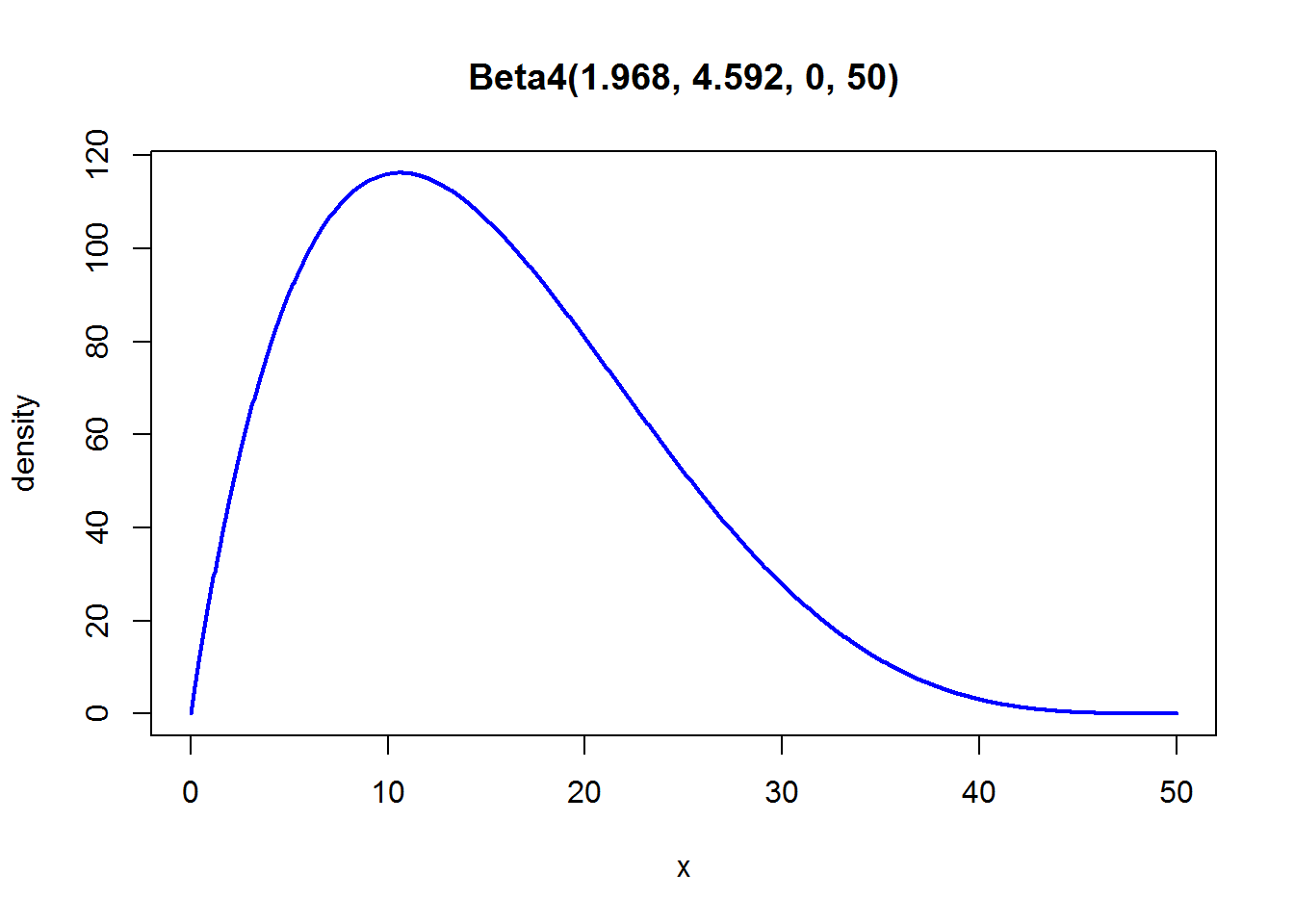the prevalence package
tools for prevalence assessment studies.

## Calculate the parameters of a Beta-PERT distribution

### Description

The Beta-PERT methodology allows to parametrize a generalized Beta distribution based on expert opinion regarding a pessimistic estimate (minimum value), a most likely estimate (mode), and an optimistic estimate (maximum value). The betaPERT function incorporates two methods of calculating the parameters of a Beta-PERT distribution, designated "classic" and "vose".

### Usage

betaPERT(a, m, b, k = 4, method = c("classic", "vose"))

## S3 method for class 'betaPERT'
print(x, conf.level = .95, ...)
## S3 method for class 'betaPERT'
plot(x, y, ...)

### Arguments

 a Pessimistic estimate (Minimum value) m Most likely estimate (Mode) b Optimistic estimate (Maximum value) k Scale parameter method "classic" or "vose"; see details x Object of class betaPERT y Currently ignored conf.level Confidence level used in printing quantiles of resulting Beta-PERT distribution ... Other arguments to pass to function print and plot

### Details

The Beta-PERT methodology was developed in the context of Program Evaluation and Review Technique (PERT). Based on a pessimistic estimate (minimum value), a most likely estimate (mode), and an optimistic estimate (maximum value), typically derived through expert elicitation, the parameters of a Beta distribution can be calculated. The Beta-PERT distribution is used in stochastic modeling and risk assessment studies to reflect uncertainty regarding specific parameters.

Different methods exist in literature for defining the parameters of a Beta distribution based on PERT. The two most common methods are included in the BetaPERT function:

Classic

The standard formulas for mean, standard deviation, $$\alpha$$ and $$\beta$$, are as follows

$mean = (a + k*m + b) / (k + 2)$

$sd = (b - a) / (k + 2)$

$\alpha = { (mean - a) / (b - a) } * { (mean - a) * (b - mean) / sd^{2} - 1 }$

$\beta = \alpha * (b - mean) / (mean - a)$

The resulting distribution is a 4-parameter Beta distribution: $$Beta(\alpha, \beta, a, b)$$.

Vose

Vose (2000) describes a different formula for :

$(mean - a) * (2 * m - a - b) / { (m - mean) * (b - a) }$

Mean and β are calculated using the standard formulas; as for the classical PERT, the resulting distribution is a 4-parameter Beta distribution: $$Beta(\alpha, \beta, a, b)$$.

Note: If $$m = mean$$, $$\alpha$$ is calculated as $$1 + k/2$$, in accordance with the mc2d package (see ‘Note’).

### Value

A list of class "betaPERT":

 alpha Parameter $$\alpha$$ (shape1) of the Beta distribution beta Parameter $$\beta$$ (shape2) of the Beta distribution a Pessimistic estimate (Minimum value) m Most likely estimate (Mode) b Optimistic estimate (Maximum value) method Applied method

Available generic functions for class "betaPERT" are print and plot.

### Note

The mc2d package provides the probability density function, cumulative distribution function, quantile function and random number generation function for the PERT distribution, parametrized by the "vose" method.

betaExpert for modelling a standard Beta distribution based on expert opinion

### Examples

## The value of a parameter of interest is believed to lie between 0 and 50
## The most likely value is believed to be 10
## .. Classical PERT
betaPERT(a = 0, m = 10, b = 50, method = "classic")
#>    method alpha  beta a  b mean   median mode      var     2.5%    97.5%
#> 1 classic 1.968 4.592 0 50   15 13.93669   10 69.44444 2.247894 33.37723
## .. Plot method
plot(betaPERT(a = 0, m = 10, b = 50, method = "classic"))## .. Vose parametrization
betaPERT(a = 0, m = 10, b = 50, method = "vose")
#>   method alpha beta a  b mean   median mode var     2.5%    97.5%
#> 1   vose   1.8  4.2 0 50   15 13.83361   10  75 1.960636 34.15672